# Kenya Certificate Of Secondary Education (KCSE 2008) Physics Paper 3

Question 1

This question consists of two parts A and B
attempt both parts.

PART A

You are provided with the following:

• copper wire
• a retort stand, boss and clamp
• an optical pin mounted on a cork
• a stop watch
• wire cutters (to be shared)
• a metre rule and a half a metre rule

Proceed as follows:

1. Clamp the cork so that the optical pin is horizontal. Hang the copper wire from the pin by the loop as shown in figure 1. Ensure that the wire is straight and the length X between the lower tip of the optical pin is 32 cm. If the length exceeds 32 cm reduce by cutting at the lower tip using the wire cutters provided.2. Displace the lower tip of the wire slightly in a plane perpendicular to the optical pin and then release it. Measure the time t for 20 oscillations of the wire and record the value in table 1.3. Repeat the procedure in (b) above for other values of X shown in the table. (Note that each length X is obtained by cutting off an appropriate length from the lower tip of the wire. For example to get X = 28 cm cut off 4 cm from the lower end.) Complete the table.

4. Plot a graph of T2(y-axis) against x. (5 marks)5.
•  Determine the slope, S, of the graph. (5 marks)

• Obtain the value of k in the equation S =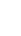(2 marks)

PART B

You are provided with the following:

• a cylindrical container
• some water
• a stop watch
• a metre rule or a half- metre rule
• a boiling tube
• some sand
• a rubber band

Proceed as follows:

1. (f) Tie the rubber band round the boiling tube so that it is at a distance L= 12 at the bottom of the tube. (see fig 2a). Pour water into the cylindrical container until the level is about 2.0 cm from the top of the beaker. Float the boiling tube in the water. Add sand gradually into the boiling tube until the tube sinks into the 12 cm mark. See figure 2(b)1. (g) Depress the boiling tube slighty and release so that it oscillates vertically without touching the sides of the container. Measure and record in the table 2 the time t1, for five oscillations of the boiling tube. Repeat the procedure two more times to obtain t2 and t3 and record the values in table 2. Complete the table.2. (h) Evaluate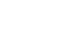given that L is the length of the tube up to the rubber band in (f) and T is the value obtained in (g) above. (2 marks)

Question 2.

This question consists of two parts A and B.
Attempt both parts.i

PART A.

You are provided with the following:

• a triangular glass prism
• a piece of soft board
• four (4) optical pins.
• a sheet of plain paper

Proceed as follows:

1. Place the plain sheet of paper on the soft board. Trace the triangular outline of the prism on the sheet of paper. Remove the prism and use a ruler to extend the three sides of the outline. See figure 3(a).Use a protractor to measure the refracting angle R on the prism.

2. On the side AB of the triangular outline, draw a normal at a point half-way between A and B. This normal will be used for the rest of the experiment.

3. Draw a line at an angle i = 30o to the normal. Stick two pins P1 and P2 vertically on this line. See figure 3(a) (5 marks)

4. Place the prism accurately on the outline. By viewing through the prism from side AC stick two other pins P3 and P4 vertically such that they are in line with the images of the pins P1 and P2. Remove the prism and the pins. Draw a line joining marks made by P3 and P4. Extend this line to meet AC. (See figure 3b)
Measure and record in table 3 the value of angle θ.5. Repeat the procedures in (c) and (d) above for the values of i shown in table 3.
Complete the table.1. On the grid provided plot the graph of the angle of emergence E (y- axis) aginst the angle of incident i. (5 marks)
2. Use the graph to find io the angle of incidence at which i = E. (1 mark)
3. Evaluate:

1. y = 2io − R (1 mark)
2. b = 2 sin i(1 mark)

PART B

You are provided with the following:

• a lens holder and a lens holder
• a screen with cross- wires
• a candle
• a metre rule

Proceed as follows:

1. Arrangle the lighted candle, the lens and the screen as shown in figure 4. Adjust the position of the screen until a sharp inverted image of the candle is formed on the screen.1. Measure the image distance v,
2. Determine the focal length of the lens using the formula.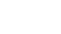(1 mark)

2. Now arrange the lighted candle the screen with cross wires and the lens as shown in figure 5. Ensure that the centre of the lens, the cross wires, and the candle flame lie on the same horizontal line. The candle flame should be placed close to the cross-wires for better illumination.1. Adjust the position of the lens until a sharp image of the cross wires is formed on the screen next to the crosswires. (Hint: You may have to rotate the lens slightly about a vertical axis so that the image of the cross wires falls on the next screen  to the cross wires and not on the cross wires).
Measure the distance d between the lens and the screen.
d = ............................................... cm (1 mark)

2. Evaluate:

1.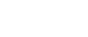(1 mark)

2.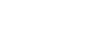(1 mark)

#### Download Kenya Certificate Of Secondary Education (KCSE 2008) Physics Paper 3.

• ✔ To read offline at any time.
• ✔ To Print at your convenience
• ✔ Share Easily with Friends / Students